# python实现鸢尾花三种聚类算法（K-means,AGNES,DBScan）

更新时间：2019年06月27日 14:44:44   作者：weixin_42134141

1.选择聚类的个数k.

2.任意产生k个聚类，然后确定聚类中心，或者直接生成k个中心。

3.对每个点确定其聚类中心点。

4.再计算其聚类新中心。

5.重复以上步骤直到满足收敛要求。（通常就是确定的中心点不再改变。

1.是解决聚类问题的一种经典算法，简单、快速

2.对处理大数据集，该算法保持可伸缩性和高效率

3.当结果簇是密集的，它的效果较好

1.在簇的平均值可被定义的情况下才能使用，可能不适用于某些应用

2.必须事先给出k（要生成的簇的数目），而且对初值敏感，对于不同的初始值，可能会导致不同结果。

3.不适合于发现非凸形状的簇或者大小差别很大的簇

4.对躁声和孤立点数据敏感

``import matplotlib.pyplot as plt import numpy as np from sklearn.cluster import KMeans from sklearn import datasets    iris = datasets.load_iris() X = iris.data[:, :4] # #表示我们取特征空间中的4个维度 print(X.shape)    # 绘制数据分布图 plt.scatter(X[:, 0], X[:, 1], c="red", marker='o', label='see') plt.xlabel('sepal length') plt.ylabel('sepal width') plt.legend(loc=2) plt.show()    estimator = KMeans(n_clusters=3) # 构造聚类器 estimator.fit(X) # 聚类 label_pred = estimator.labels_ # 获取聚类标签 # 绘制k-means结果 x0 = X[label_pred == 0] x1 = X[label_pred == 1] x2 = X[label_pred == 2] plt.scatter(x0[:, 0], x0[:, 1], c="red", marker='o', label='label0') plt.scatter(x1[:, 0], x1[:, 1], c="green", marker='*', label='label1') plt.scatter(x2[:, 0], x2[:, 1], c="blue", marker='+', label='label2') plt.xlabel('sepal length') plt.ylabel('sepal width') plt.legend(loc=2) plt.show()``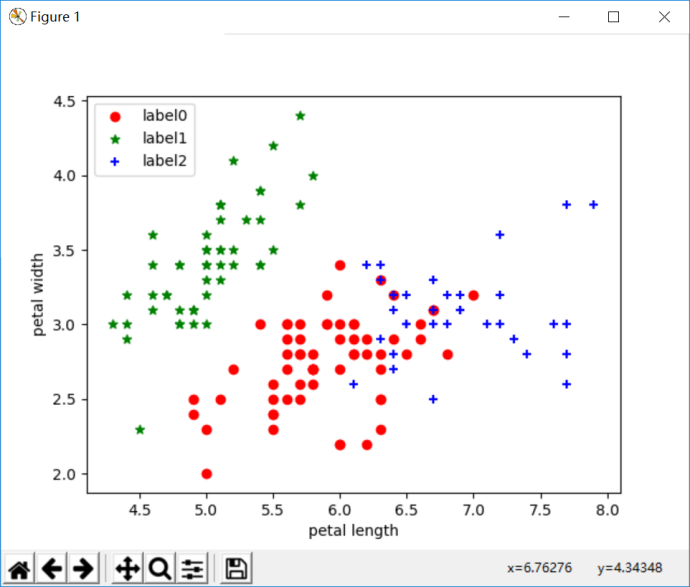二.结构性聚类(层次聚类)

1.凝聚层次聚类：AGNES算法(自底向上)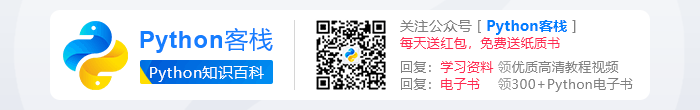2.分裂层次聚类：DIANA算法(自顶向下)

``from sklearn import datasets from sklearn.cluster import AgglomerativeClustering import matplotlib.pyplot as plt from sklearn.metrics import confusion_matrix import pandas as pd    iris = datasets.load_iris() irisdata = iris.data    clustering = AgglomerativeClustering(linkage='ward', n_clusters=3)    res = clustering.fit(irisdata)    print ("各个簇的样本数目：") print (pd.Series(clustering.labels_).value_counts()) print ("聚类结果：") print (confusion_matrix(iris.target, clustering.labels_))    plt.figure() d0 = irisdata[clustering.labels_ == 0] plt.plot(d0[:, 0], d0[:, 1], 'r.') d1 = irisdata[clustering.labels_ == 1] plt.plot(d1[:, 0], d1[:, 1], 'go') d2 = irisdata[clustering.labels_ == 2] plt.plot(d2[:, 0], d2[:, 1], 'b*') plt.xlabel("Sepal.Length") plt.ylabel("Sepal.Width") plt.title("AGNES Clustering") plt.show()``

运行结果：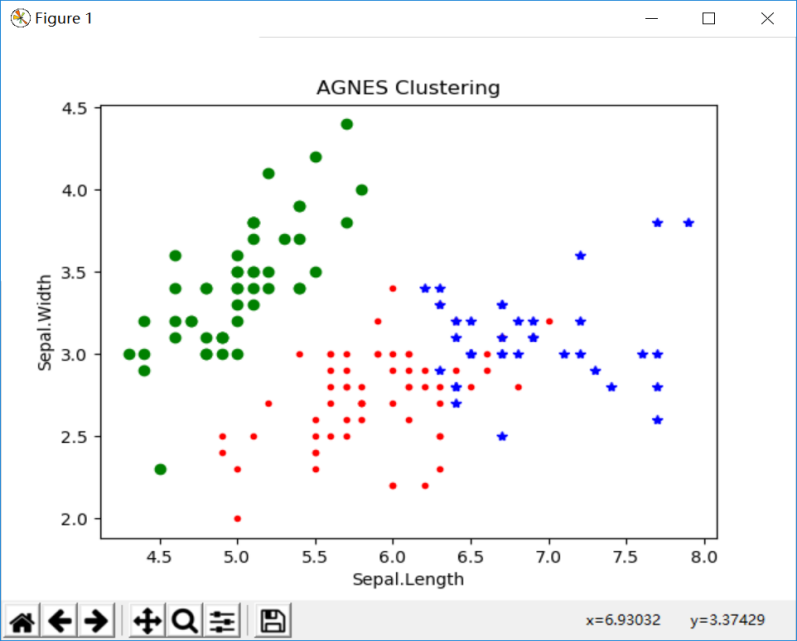``import matplotlib.pyplot as plt import numpy as np from sklearn.cluster import KMeans from sklearn import datasets from sklearn.cluster import DBSCAN    iris = datasets.load_iris() X = iris.data[:, :4] # #表示我们只取特征空间中的4个维度 print(X.shape) # 绘制数据分布图 plt.scatter(X[:, 0], X[:, 1], c="red", marker='o', label='see') plt.xlabel('sepal length') plt.ylabel('sepal width') plt.legend(loc=2) plt.show()    dbscan = DBSCAN(eps=0.4, min_samples=9) dbscan.fit(X) label_pred = dbscan.labels_    # 绘制k-means结果 x0 = X[label_pred == 0] x1 = X[label_pred == 1] x2 = X[label_pred == 2] plt.scatter(x0[:, 0], x0[:, 1], c="red", marker='o', label='label0') plt.scatter(x1[:, 0], x1[:, 1], c="green", marker='*', label='label1') plt.scatter(x2[:, 0], x2[:, 1], c="blue", marker='+', label='label2') plt.xlabel('sepal length') plt.ylabel('sepal width') plt.legend(loc=2) plt.show()``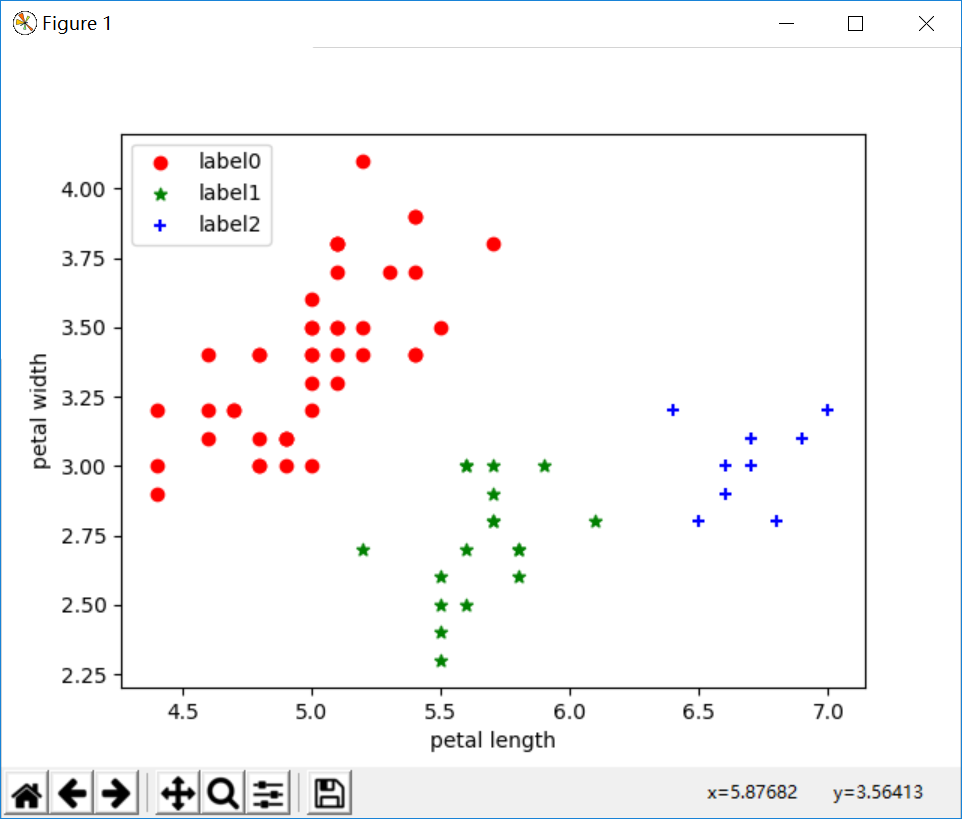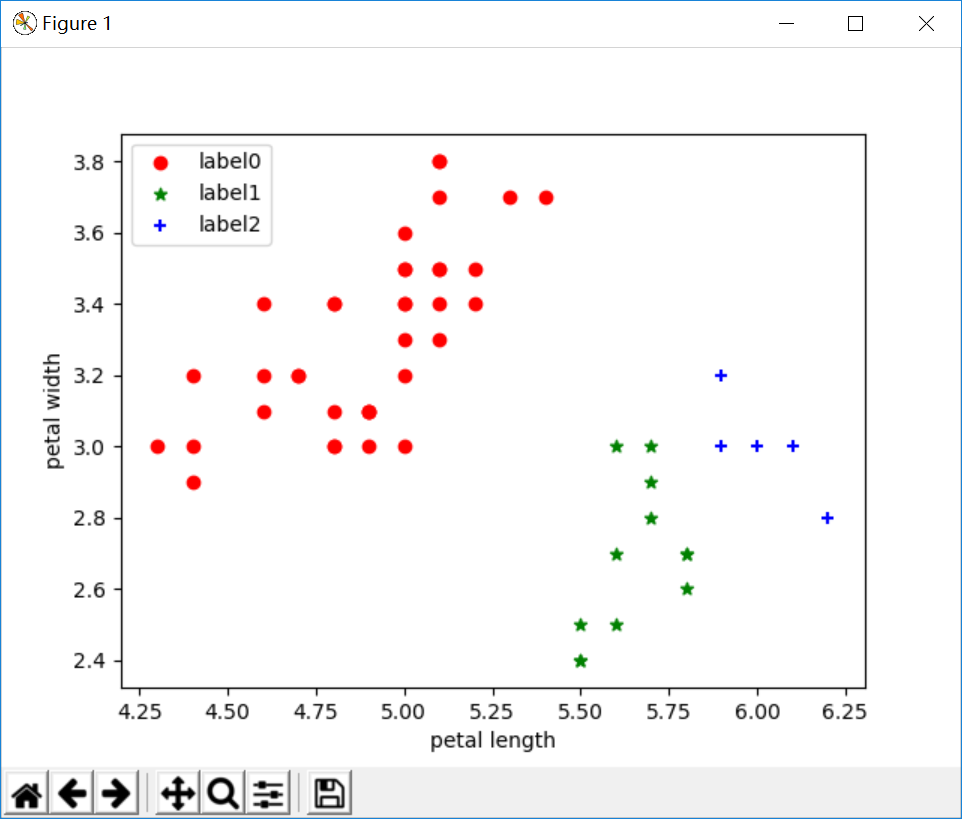k-means对于大型数据集也是简单高效、时间复杂度、空间复杂度低。 最重要是数据集大时结果容易局部最优；需要预先设定K值，对最先的K个点选取很敏感；对噪声和离群值非常敏感；只用于numerical类型数据；不能解决非凸数据。

DBSCAN对噪声不敏感；能发现任意形状的聚类。 但是聚类的结果与参数有很大的关系；DBSCAN用固定参数识别聚类，但当聚类的稀疏程度不同时，相同的判定标准可能会破坏聚类的自然结构，即较稀的聚类会被划分为多个类或密度较大且离得较近的类会被合并成一个聚类。